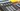BestReviews is reader-supported and may earn an affiliate commission. Details

# Best scientific calculator## Which scientific calculator is best?

Scientific calculators are ideal for complex problem-solving as well as basic arithmetic operations. Students frequently use one in math, science, engineering and statistics courses. Scientific calculators are capable of performing operations in trigonometry, logarithms and even some basic statistical analysis. They’re more functional than four-function calculators and more affordable than graphing calculators.

Scientific calculators are ideal for exams in which cellphones or advanced graphing calculators aren’t permitted. For a scientific calculator also capable of basic graphing, Texas Instruments TI-84 Plus Graphics Calculator is the top choice.

## What to know before you buy a graphing calculator

### Power source

Scientific calculators typically are powered by battery, solar power or both. Avoid devices that rely on disposable batteries, because paying for batteries every time your calculator runs out of power adds up over time. For basic scientific calculators with limited functionality, solar power is enough to power the calculator continuously. Dual power, however, gives you the peace of mind of knowing you’re backed up if the battery dies.

### Design

Scientific calculators come in a range of designs and colors. Look for a graphing calculator designed to fit easily into the palm of your hand. The model you select should have durable buttons that are easy to press. Avoid models with buttons that frequently fall off.

Calculator finishes may be glossy or matte. Glossy finishes can sometimes reflect light and be distracting, but have a clean, attractive appearance.

## What to look for in a quality scientific calculator

### Cover

If you frequently transport your calculator to and from work or class, select a model with a cover. This way, you won’t scratch the screen or dislodge the keys when you slip your scientific calculator into your pocket or bag.

### Memory

Scientific calculators with recallable memory allow you to access and edit previous strings of equations. This saves you time. You also can use this feature to look for previous mathematical errors in your calculations if you find you’ve arrived at an incorrect answer.

### Multi-function keys

Keys with multiple functions allow greater use of and functionality from your scientific calculator. You can access the second function on a multi-function scientific calculator key by pressing the “shift” or “2nd” button.

### Multi-line screen

Multi-line calculator screens let you to review previous lines of calculations for mathematical errors and to view the solution in conjunction with the operations that led to the solution. This reduces the chance of making a mistake and increases understanding of the mathematical operations you’re using.

### Undo

An undo button allows you to go back to the previous information on your calculator, undoing whatever operation you’ve just performed. This is ideal for amending a mistake without having to start a problem over from the beginning.

## How much you can expect to spend on a scientific calculator

A basic scientific calculator costs \$10-\$30. For \$20-\$90, you’ll find graphing calculators with advanced functionality, digital interfaces and graphing capabilities.

## Scientific calculator FAQ

### Do you need a scientific calculator for math class?

A. Which calculator you should buy for your math class depends on the content of the course. Scientific calculators are ideal for all basic arithmetic math courses through more complex algebraic courses. Scientific calculators also are ideal for science classes and statistics. If your course requires you to graph equations, select a graphing calculator, which performs all the functions of scientific calculators but also has the ability to plot points.

### Are scientific calculators allowed on tests?

A.  If you’re taking an exam for a course, check with your instructor about what, if any, calculators are permitted at the exam. Standardized tests such as the SAT permit scientific calculators.

### How hard is it to learn to use a scientific calculator?

A. How much time it will take you to gain mastery of your scientific calculator’s functions depends on the range of functionality of your scientific calculator. Basic arithmetic operations are straightforward, but more advanced algebraic functions take some time to figure out. Look for a scientific calculator with high ratings for usability.

## What’s the best scientific calculator to buy?

### Top scientific calculator

Texas Instruments TI-84 Plus Graphics Calculator

What you need to know: This is a graphing calculator with all scientific-calculator functions built in from a trusted brand ideal for basic math and algebra.

What you’ll love: Ideal for serious math students who want a scientific calculator with an advanced interface, it’s also useful for students in courses including chemistry, biology and statistics. It has graphing capability and easily navigable menus.

What you should consider: This calculator costs a good deal more than the average scientific calculator.

Where to buy: Sold by Amazon

### Top scientific calculator for the money

Casio fx-115ES PLUS Engineering/Scientific Calculator

What you need to know: This is a scientific calculator with both battery and solar power at a good price.

What you’ll love: With a library of 280 functions and the ability to perform operations on fractions, this scientific calculator works well for most basic math courses. It is approved for use on many standardized exams.

What you should consider: The battery life on this calculator is short.

Where to buy: Sold by Amazon

### Worth checking out

Sharp EL-501XBGR Scientific Calculator

What you need to know: This is a good scientific calculator with a range of basic functions at a price you’ll like.

What you’ll love: It is an affordable, attractive, glossy plastic calculator with easy-to-read buttons. It’s small enough to fit nicely into a bag for transportation. It is good for students who need to perform basic arithmetic and algebraic operations.

What you should consider: Some users find the functions on this calculator counterintuitive to use.

Where to buy: Sold by Amazon